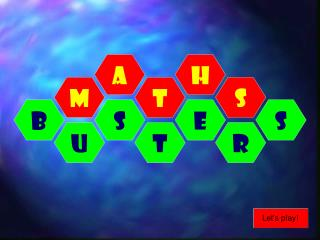Download PresentationWhat A?What A? - PowerPoint PPT Presentation

Download PresentationWhat A?
An Image/Link below is provided (as is) to download presentation

Download Policy: Content on the Website is provided to you AS IS for your information and personal use and may not be sold / licensed / shared on other websites without getting consent from its author. While downloading, if for some reason you are not able to download a presentation, the publisher may have deleted the file from their server.

- - - - - - - - - - - - - - - - - - - - - - - - - - - E N D - - - - - - - - - - - - - - - - - - - - - - - - - - -
Presentation Transcript

1. A H S M T B S E S U R T

2. A Z F T E S F N F P I B Z O C T S E D O F M S O N

3. What A? • What A is the ‘A’ in BIDMAS? • Addition

4. What S? • What S is 3 x 5 + 2? • Seventeen (17)

5. What I? • What I is the ‘I’ in BIDMAS? • Indices (Index)

6. What T? • What T is 4 x 3 + 7 x 2? • Twenty-six (26)

7. What F? • What F is 42 – 1? • Fifteen (15)

8. What T? • What T is (7 – 4) x 4? • Twelve (12)

9. What F? • What F is (12 – 5) x (5 + 3)? • Fifty-six (56)

10. What O? • What O is 10 x (12 + 6)? • One-hundred-and-eighty!! (180)

11. What D? • What D is the opposite of multiplication and the ‘D’ in BIDMAS? • Division

12. What O? • What O is 3 x 5 – 7 x 2? • One (1)

13. What Z? • What Z is 1 + 4 ? 10 • Zero point five (0.5)

14. What F? • What F is (3 + 3) x 7 + 1? • Forty-three (43)

15. What B? • What B is the first operation in BIDMAS? • Brackets

16. What S? • What S is to multiply a number by itself? • Square

17. What M? • What M is the inverse of division and the ‘M’ in BIDMAS? • Multiplication

18. What E? • What E is 22 x 2 + 3? • Eleven (11)

19. What P? • What P can mean ‘out of 100’? • Percentage

20. What C? • What C means to multiply a number by itself, then by itself again? • Cube

21. What O? • What is 1 + 10 x 10? • One-hundred-and-one (101)

22. What N? • What N is 5 x 2 + (3 x 3)? • Nineteen (19)

23. What F? • What F is (6 + 2) x 5? • Forty (40)

24. What N? • What N is 6 x 3 - 9? • Nine (9)

25. What Z? • What Z is 9 – 3 x 3? • Zero

26. What E? • What E is 9 x (2 + 7)? • Eighty-one (81)

27. What S? • What S is 7 x (10 + 1)? • Seventy-seven (77)